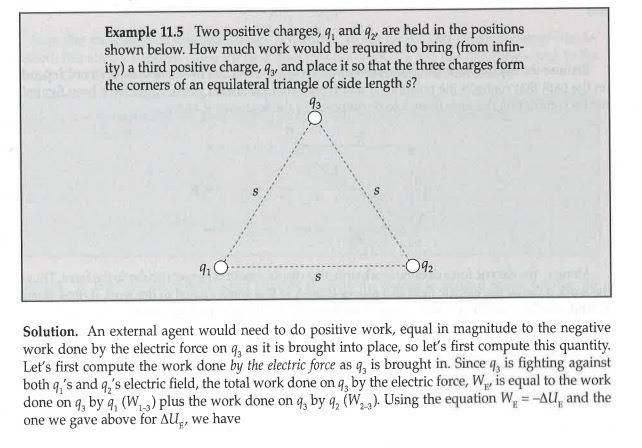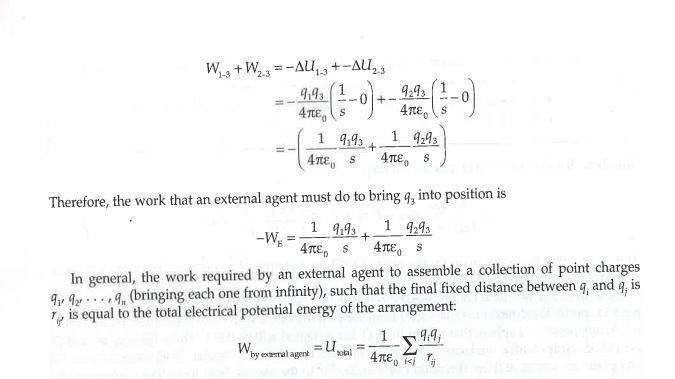# Question on finding work done on charge

## Homework Statementcoulomb's law

## The Attempt at a Solution

Hi everyone. I understand their approach with the integration to find the amount of work that "a person" would have to do to bring the charge q3 from infinity to its current position.

I understand that the force that this person would have to do is the opposite of the coulombic forces between the q3 and q1 and 2.

My question: why did they not account for the y-components of the coulombic forces between q1 and 2 to a3? The x-components of the two forces would cancel, so the only coulombic forces acting on q3 would be the y-components.

Thus I did exactly what they did, but I added a sine term to account for the y-component and then re-wrote the sine in terms of the vertical distance and "s/2" with pythagorean theorem and then did the integration.

Could anyone please weight in on why their approach is legit and they did not do what I planned to do with the sine term?

Thank you very much in advance.[/B]